International Journal of Geomagnetism and Aeronomy
Vol. 2, No. 3, September 2001

# Accretion of magnetized plasma on a gravitational center

S. A. Dyadechkin and V. S. Semenov

Physical Institute, St.-Petersburg University, St.-Petersburg, Russia

H. K. Biernat

Space Research Institute, Austrian Academy of Sciences, Graz, Austria

### Abstract

The magnetic field frozen into protoclouds appreciably affects the process of formation and evolution of young stellar objects (YSO). Many effects in the vicinity of YSO are associated with the magnetic field (e.g., bipolar flows, optical jets). In the description of magnetohydrodynamic (MHD) phenomena, the concept of a magnetic flux tube frozen into the plasma flow often plays an important role. We use here a method of introducing Lagrangian coordinates into the MHD equations, which enables a convenient mathematical formulation for the consideration of the behavior of a magnetic flux tube. With the introduction of a Lagrangian coordinate system co-moving with the flux tube, the MHD equations of motion reduce to nonlinear string equations. Therefore, the behavior of flux tubes can be studied through solving these string equations. The behavior of a string and a free particle in the gravitational field are very different. The gravitational center can never capture a free particle if the particle has a non-zero impact parameter, the string, on the contrary, can be captured. Using a numerical simulation for solving the MHD equation system in the vicinity of the gravitational center, we found out that two different kinds of motion exist. The first is capture of the string by the gravitational field, and the second is free string motion. We investigated also the influence of the reconnection process on the string motion in the vicinity of the gravitational center. It tuned out that the reconnection process can change the kind of string motion.

### Introduction

It is well known these days that a star originates as a result of the collapse of interstellar clouds of magnetized molecular gas. Thus, the process of star formation depends on the properties of a protostellar cloud, and for the understanding of this process we need to consider the accretion of magnetized plasma on a gravitational center. At some stage, the magnetic field becomes strong enough to modify profoundly the dynamics of gravitational contraction, in particular, by introducing an essential anisotropy into the problem [Mestel, 1985]. This makes, generally speaking, the problem a time-dependent and three dimensional one.

A similar situation occurs in many problems of space physics where the magnetic field strongly influences the plasma motion, for example, in the case of solar wind-magnetosphere interactions [Biernat, 1987; Biernat et al., 1991]. In this case a relatively weak interplanetary magnetic field is amplified more than ten times in the course of solar wind flow around the magnetosphere, producing a so-called magnetic barrier or depletion layer [Erkaev, 1989; Zwan and Wolf, 1976]. It turns out that such a problem with strong magnetic field can be successfully solved using the thin flux tube approximation. The general idea of this approach is quite simple: We have to take a test flux tube and let it go with the solar wind in the case of the magnetosphere, or under influence of gravitation in the case of star formation. The time evolution of this test flux tube can provide a clear physical description of a complicated 3D time-dependent plasma flow.

For example, for the Earth's magnetosphere such an approach gives the famous Dungey model [Dungey, 1961], which can describe many important features of the solar wind-magnetosphere coupling:

(1) The magnetic barrier (depletion layer) formation.

(2) Dayside magnetopause reconnection.

(3) Flux transfer from the dayside magnetosphere to the nightside.

(4) The growth phase of a magnetospheric substorm (magnetic energy accumulation in the magnetotail).

(5) Reconnection in the magnetotail (magnetospheric substorm).

Of course, we can not apply directly the Dungey model to the completely different process of plasma accretion on a gravitational center. Instead, we can make the following general conclusions which we have to take into account for other applications:

(1) In a first approximation, the solar wind-magnetosphere coupling can be described in terms of flux tubes.

(2) The inhomogeneous motion of space plasmas leads to the accumulation of Maxwellian tensions (magnetic energy).

(3) The relaxation of accumulated Maxwellian tensions is achieved through magnetic reconnection.

(4) Magnetic reconnection is initiated inside a small diffusion region as a result of the development of anomalous resistivity.

So, our idea is the following: We will consider the motion of a test flux tube in the vicinity of a gravitational center similar to the classical investigation of the motion of a test particle.

### The MHD Equations

From a mathematical point of view, in order to obtain the time evolution of a flux tube, we first of all need to formulate the appropriate equations. To this end we will introduce Lagrangian coordinates into the MHD equations to obtain a convenient mathematical formulation for the flux tube motion [Semenov and Erkaev, 1989].

The following system of MHD equations (in gaussian units) describes the plasma behavior in the vicinity of a gravitational center:(1)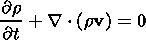(2)(3)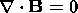(4)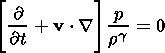(5)

Equation (1) is the equation of motion, equation (2) is the continuity equation, equation (3) is the induction equation (in the limit of infinite conductivity, i.e., Rem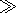1, where Rem is the magnetic Reynolds number), equation (4) encapsulates the solenoidity property of the magnetic field, and equation (5) is the equation of state. Here r, v, p, B, y, r, g are the density, the velocity, the plasma pressure, the magnetic field, the gravitational potential, the space coordinate, and the ratio of specific heats, respectively.

Using dimensionless variables,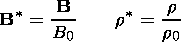the system of equations (1)-(5) can be rewritten as (we shall omit the "*" from now on):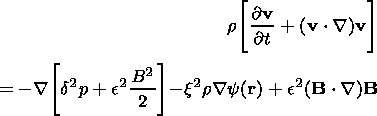(6)(7)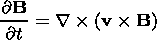(8)(9)(10)

The parameters, x and c are defined as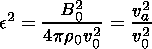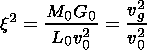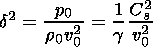(11)

where va and Cs are Alfvén and sound speed respectively.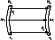Figure 1

### Frozen-in Coordinate System

The equation of motion (6) describes the behavior of a magnetic flux tube in the gravitational field. To appreciate this more fully, we have to rewrite (6), using a Lagrangian coordinate system (i.e., a coordinate system co-moving with the magnetic flux tube).

The following conservation laws are satisfied in the process of the magnetic flux tube motion (Figure 1):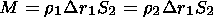(12)

the mass conservation law,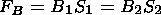(13)

and the flux conservation law. Therefore using (14) and (15) we can introduce a new variable a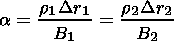(14)

Thus, a is the mass of the magnetic flux tube with unit flux, and consequently, we can measure the length of a tube in units of mass. Another variable which we use is the Lagrangian time t (i.e., motion particle time along the trajectory). In this case it can be shown that [Semenov and Erkaev, 1989]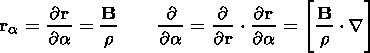(15)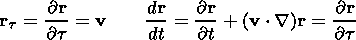(16)

consequently,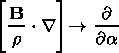(17)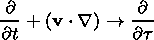(18)

Furthermore, we can use (15)-(18) and rewrite the equation of motion (6) in frozen-in coordinates. Thus, we derive the following system of equation: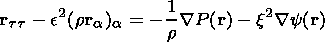(19)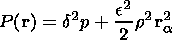(20)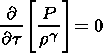(21)

The MHD equation of motion (6), written in frozen-in coordinates is the equation of a non-linear string, which is well known in mathematical physics and plays an important role in the description of many wave-like processes. The left-hand side of equation (19) has the form of the 1D non-linear string equation and the right-hand side incorporates the effects of the total pressure and gravitational potential. Therefore, we can establish an analogy between the non-linear string and the magnetic flux tube. This description is very convenient for various problems, because often it gives important physical insight [Erkaev, 1989]. In our case, it is more convenient to consider an isolated magnetic flux tube.

There is another way to bring out the analogy between the magnetic flux tube and the non-linear string. This can done by employing the variational method.

We can write the Lagrangian L for the magnetic flux tube as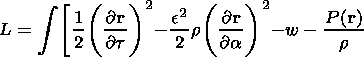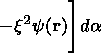(22)

where w is the internal energy of the plasma, w=d2k[rg-1/ (g-1)]. The Hamiltonian H is written as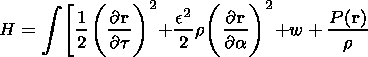(23)

Furthermore, we can use the variational method and derive the system of MHD equations in frozen-in coordinates (19)-(20).

Also, we can extract a useful property from the Hamiltonian (23). Integrating with respect to time we have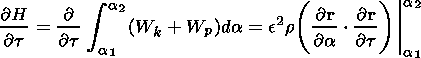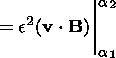(24)

where Wk and Wp are the kinetic and potential energy respectively,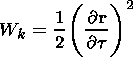(25)(26)

From (24) we can see that the variation of the total energy in the magnetic flux tube is equal to the flux through the ends.

To investigate the behavior of a magnetic flux tube falling towards a gravitational center we solve the system of equations (19)-(21) using a Lax-Wendroff numerical method. This is a two-step method which is often used for the solution of similar problems.

### Results of the Numerical Simulation

The motion of a free particle is well known [Landau and Lifschits, 1988]. In a gravitational center, a free particle moves along Kepler orbits, and if a particle has a non-zero impact parameter it never can be captured by a gravitational center. For the particles in the magnetic flux tube, the motion is evidently different from that of a free particle, because the magnetic field will put a brake on the tube element (particle). Thus, as it loses angular momentum and energy, a particle will change its orbit gradually, approach the gravitational center, and finally it will be captured.Figure 2
As initial conditions for the numerical simulation we take an undisturbed magnetic flux tube with length l, a distance to the gravitational center of L, and an impact parameter P (Figure 2). Quantities L, l and P are supposed to satisfy the following conditions(27)

Furthermore we perform a parametric investigation of the string behavior, varying parameters, x and c and considering their influence on the string motion.

We obtain the following results. There are two different kinds of motion of the magnetic flux tube in the vicinity of the gravitational center: capture of the string, and free string motion.

In the case of the string capture (Figure 2), the central part of the flux tube begins to fall towards the gravitational center faster than distant parts. The closer points of the string are situated to the gravitational center, the faster they move. Thus, the distance between the central part and other parts of the flux tube increases and the flux tube is strongly stretched towards the gravitational center. The numerical simulation in this case is continued up to the moment when the central point of the string reaches a small region around the gravitational center.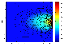Figure 3
In Figure 3 we see the density behavior in this case. During the string motion, the density increases in the central part of the flux tube. The plasma from distant regions of the flux tube flows towards the central part of the string under the influence of the gravitational center. The more mass flows into the central region of the flux tube, the stronger the influence of the gravitational center on the unit element of the central part of the string. Thus, the central part of the string falls onto the gravitational center faster and faster until it is finally captured. Evidently, the magnetic field in the string is increased as a consequence of the stretching of the flux tube.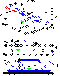Figure 4
The second case is the free string motion (Figure 4).

In the case of a free string motion, the influence of the gravitational field on the flux tube is less pronounced than in the previous case. In this case the gravitational field does not capture the string, but changes the direction of the flux tube motion (Figure 4). At first the string is strongly stretched under the influence of the gravitational center, as in the case of string capture. However, after passing through the immediate neighborhood of the gravitational center, the shape of the string recovers the initial undisturbed configuration.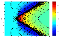Figure 5
In the case of the free string motion, the density behavior is similar to the case of string capture at first. However, after passing the closest point to the gravitational center, the character of the motion changes. Under the influence of the gravitational field, two oppositely directed MHD waves are generated in the string (Figure 5). They move along the flux tube from the central part to the distant regions of the string and modify the density in the flux tube. The string density increases after passage of the waves. As a result, the density along the string increases and the string, as a whole, is compressed (Figure 4). It is clear why such an effect takes place, because the mass conservation law has to be satisfied in the magnetic flux tube.

Thus, in this case, the gravitational center influences the string similar to the influence of a displacement along an infinite string. It is well known that after the displacement, an infinite string just changes its position without a change of shape. In the case of the free string motion we have a similar effect. But in our case the gravitational center changes the direction of the string motion and density in the flux tube.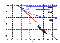Figure 6
It is well known that if the free particle has a non-zero impact parameter, it can never be captured by the gravitational center. However, the string can be captured. Thus, there is a strong difference between the free particle motion and the motion of string particles. This difference is the result of the magnetic field influence on the particles in the flux tube. In Figure 6 we can see that even a weak magnetic field has a strong influence on the motion of flux tube particles.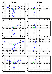Figure 7
The reconnection process can radically change the kind of motion of the magnetic flux tube in the gravitational field. During the string motion, there is the possibility of the appearance of a flux tube configuration like in Figure 7a. Different parts of the magnetic flux tube with oppositely directed magnetic field are situated close to each other. We note that the approach of a single flux tube is rendered invalid at this moment. A detailed consideration of conditions for the initiation of reconnection is out of the frame of this study.

We assumed that the reconnection process occurs at this moment. As a result, the string is divided into two parts. The first part rapidly falls towards the gravitational center, and the second part moves away.

### Conclusions

(1) The behavior of magnetic flux tube is radically different from free particle motion. The string with non-zero impact parameter can be captured by a gravitational center. However, the free particle with a similar parameter will never be captured. Thus, there are two types of string behavior in the vicinity of the gravitational center: Capture of the string, and the free motion of the string.

(2) In the case of the free string motion, two oppositely directed waves are formed in the flux tube, which propagate from the central part of the string to distant regions of the flux tube and increase the string density.

(3) The reconnection process has a major influence on the string behavior and can change the kind of string motion.

#### Acknowledgment

This work is supported by the INTAS-ESA project 99-01277, by the INTAS project YSF-80, by the Programme of the Ministry of High School "Intergeophysics'', and by the Austrian "Fonds zur Förderung der wissenschaftlichen Forschung'' under project P13804-TPH. We acknowledge support by the Austrian Academy of Sciences, "Verwaltungsstelle für Auslandsbeziehungen.''

### References

Biernat, H. K., Coupling processes at the magnetopause, in Theoretical Problems in Space and Fusion Plasma, pp. 105-118, Graz, 1991.

Biernat, H. K., M. F. Heyn, and V. S. Semenov, Unsteady Petschek reconnection, J. Geophys. Res., 92, 1987.

Dungey, J. W., Interplanetary magnetic field and the auroral zones, Phys. Rev. Lett., 6, 47, 1961.

Erkaev, N. V., A solar wind flowing over the Earth's magnetosphere, in Solar Wind-Magnetosphere Interaction, Results of Researches on the International Geophysical Projects, Moscow, 1989.

Landau, L. D., and E. M. Lifschits, Mechanics, Moscow, 1988.

Mestel, L., Magnetic Field, Protostars and Planets II, pp. 320-339, 1985.

Semenov, V. S., and N. V. Erkaev, Non-linear strings, Geomagn. Aeron. (in Russian), 29, 222, 1989.

Zwan, B. J., and R. A. Wolf, Depletion of solar wind plasma near the planetary boundary, J. Geophys. Res., 81, 1636, 1976.

Load files for printing and local use. This document was generated by TeXWeb (Win32, v.1.3) on October 10, 2000.